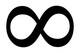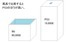# Unresolved Detail In Plotted Equations

Sometimes the calculator detects that an equation is too complicated to plot perfectly in a reasonable amount of time. When this happens, the equation is plotted at lower resolution.

267 out of 297 found this helpful
Have more questions? Submit a request

•Kyle Drang

try this one

\frac{d\tan \left(x^2\right)}{g\tan \left(a^2\right)}+\frac{f\tan \left(y^2\right)}{h\tan \left(b^2\right)}\ \ge 1

•Neeleyl9565

I'm back.

Stairs: \operatorname{floor}\left(\operatorname{ceil}\left(x\right)\right)=\operatorname{ceil}\left(\operatorname{floor}\left(y\right)\right)

kewl stripes: \sin \left(\cos \left(\tan \left(\csc \left(\sec \left(\cot \left(\operatorname{floor}\left(\operatorname{ceil}\left(xy\right)\right)\right)\right)\right)\right)\right)\right)>\frac{\operatorname{floor}\left(\frac{x}{y}\right)\operatorname{ceil}\left(\frac{y}{x}\right)}{xyxy}

A tear in space: 10\le \operatorname{floor}\left(\frac{x}{y}\right)yx

•Raffy Tabingo

lcm(x,y)/gcf(x,y) = 0

•Matthew Hardesty Jr

Sqrt(2+x)•sqrt(2+x)=2+x
Is pretty odd.

•Chethanmachandra

Try

f(y) = -sin(y) (Turn off color)

f(xy) = sin(xy)

•Neeleyl9565

I destroyed Desmos using memes.

\cot \left(now\cdot this\cdot is\cdot a\cdot story\cdot all\cdot about\cdot how\cdot my\cdot life\cdot got\cdot flipped\cdot turned\cdot upside\cdot down\right)=x

Be sure to click "all".

•Leo Needleman

\cos \left(\sin \left(\tan \left(\frac{\sin \left(\tan \left(x!^{\cos \left(\frac{x}{\tau !\pi !}\right)}\right)\right)}{\sin \left(\cos \left(\left|\tan \left(\frac{\pi !}{y}\right)\right|\right)\right)}\right)\right)\right)^2=\sin \left(\cos \left(\tan \left(\frac{\cos \left(\tan \left(y!^{\left(\sin \left(\frac{y}{\sqrt{\tau \ }\sqrt{\pi }}\right)\right)}\right)\right)}{\cos \left(\sin \left(\left|\tan \left(\frac{\pi !}{x}\right)\right|\right)\right)}\right)\right)\right)^2

Wow. Really big.

•Wombatnaynay2

The fact I found this one in eighth grade makes me confused

https://www.desmos.com/calculator/ikkrav7l2z

•Neeleyl9565
•Lee Zao

and also y = root x *mod(x,y)

•Chethanmachandra

MWAHAHAAAAAA DESMOS WILL DIE UNDER MY COPY AND PASTING OF THE MAJORITY OF THE COMMENTS ON THE FIRST PAGE!!!!!!! DIE DESMOS!!!!

seriously, I copied and pasted a bunch of your suggestions onto this one evil graph, I also added a rose curve where the variable is always changing

It may take a while to load, it's so slow with all the graphing insanity

Edited by Chethanmachandra
•creepercraft97T3

I made an equation that gives you 2 numbers: x and y, but x is exactly 1 higher than y. I added a plus one to the end of it and got an INSANE graph with dots and lines EVERYWHERE! The weird thing, though, is the space that is almost completely empty. So weird.

Well, anyways, here is the equation

x/(x-y)=y/(x-y)+1

<!> WARNING: <!>

Because of how insane the graph is, it is EXTREMELY LAGGY!!!

Here is a link to the graph: (I deleted graph as I made another with 3 more versions of the same equation below)

Edited by creepercraft97T3
•creepercraft97T3

I have 3 more graphs already! LOL!!! (I wonder if I will take this forums over like the cool commands minecraft forum)

Anyway, here are the graphs:

#1: EQUATION: sqrt(sin(x))=cos(y)   GRAPH: https://www.desmos.com/calculator/jpo5mzxise

#2: EQUATION: sin(x)*cos(y)=0   GRAPH: https://www.desmos.com/calculator/mc4efuuvhb

#3: (This one doesn't have a graph because it kills DESMOS)

EQUATION: (x^x^x/x^x^y/x^y^x/x^y^y/y^x^x/y^x^y/y^y^x/y^y^y)=0

Edited by creepercraft97T3
•Henry Lee

(xy)!!(y!!)^(x!!)=(xy)!(x!!)^(y!!)

is a good one

•Amerus of Mairon

(x^2-y^2)^2+(2xy)^2=(x^2+y^2)^2

•Brycehawken

y=\tan \left(\operatorname{abs}\left(\frac{\log \left(x\right)}{\log \left(y\right)}\right)\right)

Rather cool

•Marcbartolabac130243

Solve this and I will give you a NOBEL PRIZE:

Show that:

(d/dx((x-1)!)=Σ(y on top,n=0 below)nx^(n-1)

•Marcbartolabac130243
•creepercraft97T3

I made SEVEN more variations of the equation and put them all in one graph. It gives you a complete picture of what the graph would look like.

I will not be posting the equations here, sadly.

This is the graph: https://www.desmos.com/calculator/v5lch8jjqb

The line represents the places the equation IS NOT true.

(I redid this entire post, just saying)

Edited by creepercraft97T3
•伊藤那由多

@Marcbartolabac130243

It was actually impossible to solve.

First, we have to check how each part of the expression is interpreted in Desmos.

d/dx means derivative, as we know.

x! seems to be interpreted as Γ(x+1), so the (x-1)! on the left side is actually Γ(x).

Therefore, the left side actually says Γ'(x).

Next, the right side. We have to determine what fractional sigma means. (How can we calculate the right side if y=2.3?)

With some experiments, I discovered that fractional number above or below sigma is actually rounded to the nearest integer. For example, if y=2.3, the sum adds up n=0, n=1, and n=2.

Now the problem gets completely interpreted to an infinite set of the equations:

Solve Γ'(x)=0 for x.

Solve Γ'(x)=1 for x.

Solve Γ'(x)=2x+1 for x.

Solve Γ'(x)=3x^2+2x+1 for x.

Solve Γ'(x)=4x^3+3x^2+2x+1 for x.

...

Obviously, this is impossible to solve analytically.

And my graph is:

\sin xy=\tan xy

•creepercraft97T3

x/(x-y)=y/(x-y)+1

•Marcbartolabac130243

Nope.
(thanks asian guy)
(sorry for being racist)
Anyway...
I think I solved your laggy equations!😁
I also know why desmos lags here.
Here, I replaced all the Xs with As and Ys with Bs on the left side.
I made it self explanatory in the graph so...
What else to...

https://www.desmos.com/calculator/imkwri5lq1

Trust me
YOU WILL BE IMPRESSED!

•David Robillard

\tan y^{y^y}=\tan x^{x^x}

•Allison36382

fix it then!!!

•Cldonkin

I made this for a project in PreCalc sophomore year in an attempt to use the flower in a project but honestly its just fun to watch

•Cldonkin

i spend way too much time going through this forum

have another graph!

edit

i've already posted this graph here, who knew? clearly not me...

Edited by Cldonkin
•David Robillard

\operatorname{mod}\left(y^x,x^y\right)=\operatorname{mod}\left(x^y,y^x\right)

\cos \left(y^xx^y\right)=\sin \left(y^xx^y\right)

•David Robillard
•Roxas Hikari (ロクサス)

15\sin \left(\frac{65x^4-81x^3+2x^2-52x-250}{343x^3-3x^2+3x+15\left(.056\right)^{3x\left(\cos \left(54y-3x^y\right)\right)}}\right)=\frac{y\sin \pi ^x}{3}

•GD TheProGamer711

Hmmmmmmmmm...